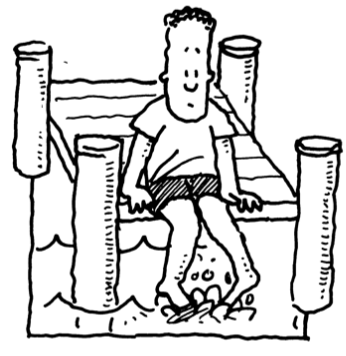### Home > PC > Chapter 9 > Lesson 9.3.4 > Problem9-146

9-146.

Otis, from Redding, was sitting at the dock of a crystal bay wasting time. As he was sitting in the morning sun watching the tide roll away, he took hourly readings of the water level on one of the posts holding up the dock. Ever since he had left his home in Georgia he was interested in trigonometric functions, so he decided to find a mathematical model to approximate the water level in feet at any time $t$. Otis was a little depressed and hence was having trouble finding an equation of the curve of best fit.Time

Water Level

Time

Water Level

Time

Water Level

5 AM

$17.82$

1 PM

$18.04$

9 PM

$10.38$

6 AM

$15.63$

2 PM

$19.49$

10 PM

$10.70$

7 AM

$12.54$

3 PM

$20.00$

11 PM

$12.89$

8 AM

$11.19$

4 PM

$19.87$

12 Midnight

$15.42$

9 AM

$10.20$

5 PM

$17.77$

1 AM

$18.04$

10 AM

$10.96$

6 PM

$15.55$

2 AM

$19.53$

11 AM

$13.14$

7 PM

$13.15$

3 AM

$20.35$

12 Noon

$15.17$

8 PM

$10.82$

4 AM

$19.74$

1. Help Otis out of the blues by finding a trigonometric model that approximates his data. Choose convenient values for the water level.

Convenient range would be $[10,20]$. Therefore, vertical shift would be $15$.

$y = 15-5\text{sin}\left( \frac{\pi}{6} (x-1) \right)$

2. Otis’ girlfriend wants to believe that he was really at the dock of the bay so she asks him what the water level was at 7:30 PM. Help Otis save his relationship by using your model to find the water level at that time.

$11.46$ feet

3. While Otis was sitting he noticed the water went past the $14$-foot mark several times. Using your model, find all of the times (in the first $24$ hours) when the water reached the $14$-foot level.

$x = 1.382$
x = 5 AM + 1 hour, 23 minutes = 6:23 AM
Other values = 11:37 AM, 6:23 PM, 11:37 PM

Use the eTool below to see the data at each hour.
Click the link at right for the full version of the eTool: 9-146 HW eTool